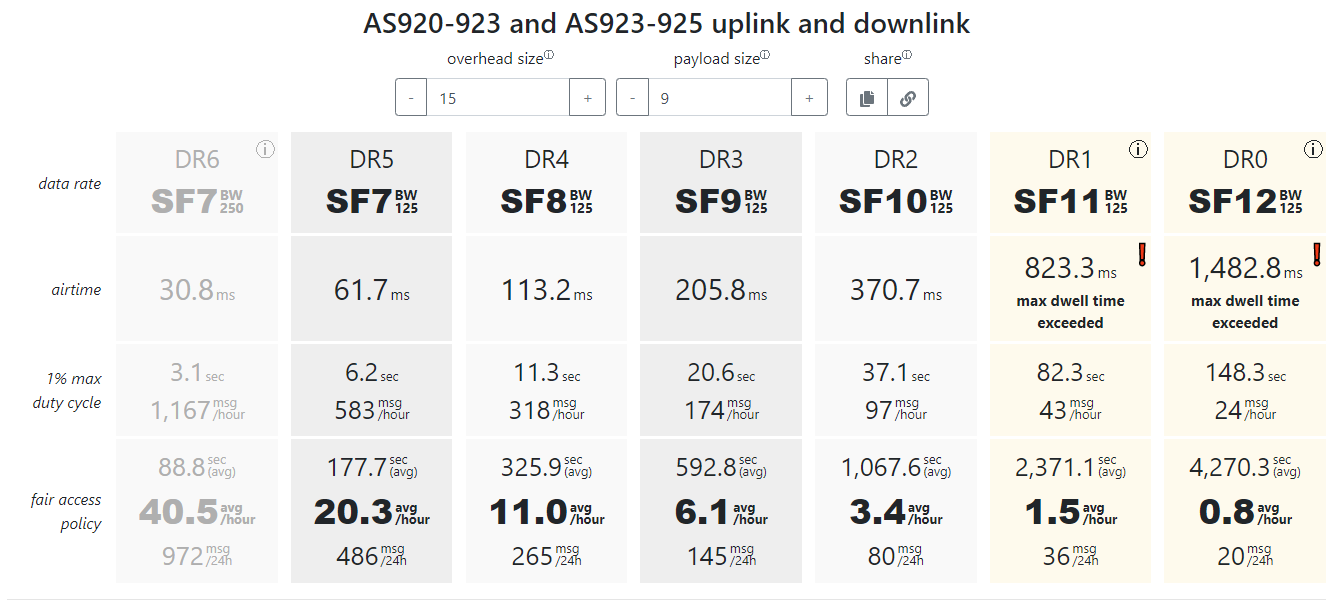# What is maximum number of sending payload at AS923?

• Hello, I've tried to send sensor data using Lopy4 at AS923, I'm using Thethingstack network server and kerlink gateway. It can send maximum only 9 byte data at SF10, and then I check in lorawan airtime calculator (https://avbentem.github.io/airtime-calculator/ttn/as923/) the overhead size in lopy4 uplink is 15 byte..

I've tried to send 2 payload at SF7 for each 1 hour and it is successfully received in network server and application server. and then I tried to send 10 payloads with 60 second each payload.,
but after that the uplink from mylopy is can't be received in network server until waiting 5 hours. what caused this issue to happen?

• @Gusti-Made-Arya-Wijaya I using downlink to trigger lopy4 to send payload data that saved in .txt file, here is the code

``````def new_req_log(self):
try:
print('fungsi new_req_log')

print(targetfile)
print(targetmsg)
cari_tgl=(''.join(['{:02x}'.format(b) for b in targetfile]))
print(cari_tgl)
print('Request Log tanggal '+ cari_tgl)

print('selecting file')
file_log = os.listdir('log')
file_log.sort(reverse=True)

c = 0
targeted = 0
for a in range(len(file_log)):

c = c
cari = file_log[a]
target = cari[4:8]

if target == cari_tgl:

targeted = c

c = c + 1

with open('log/' + read_log_last) as f:

for n in range(len(targetmsg)):
i = int.from_bytes(targetmsg[n:n+1], 'big')
data = content[i].replace('FF04', 'FF05')  # log data id
log_data = ubinascii.unhexlify(data.replace('\n', ''))  # convert string log to byte
print(log_data)